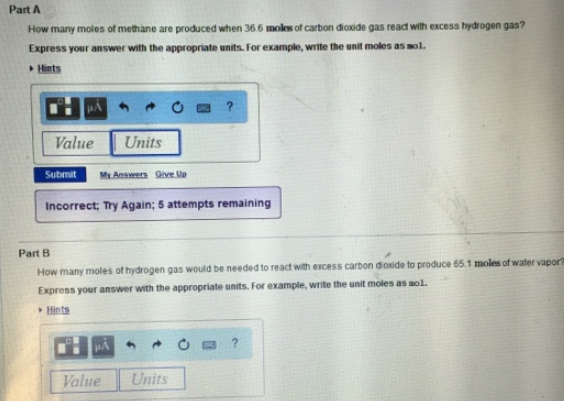# Problem: Part AHow many moles of methane are produced when 36.6 moles of carbon dioxide gas read with excess hydrogen gas? Express your answer with the appropriate units. For example, write the unit moles as mol. Part BHow many moles of hydrogen gas would be needed to react with excess carbon dioxide to produce 65.1 moles of water vapor? Express your answer with the appropriate units. For example, write the unit moles as mol.

###### FREE Expert Solution
92% (205 ratings)###### Problem Details

Part A

How many moles of methane are produced when 36.6 moles of carbon dioxide gas read with excess hydrogen gas? Express your answer with the appropriate units. For example, write the unit moles as mol.

Part B

How many moles of hydrogen gas would be needed to react with excess carbon dioxide to produce 65.1 moles of water vapor? Express your answer with the appropriate units. For example, write the unit moles as mol.